# CSMA CD Protocol | CSMA CD Flowchart

## Access Control in Networking-

Before you go through this article, make sure that you have gone through the previous article on Access Control.

We have discussed-

• Access Control is a mechanism that controls the access of stations to the transmission link.
• Broadcast links require the access control mechanism.
• There are various access control methods-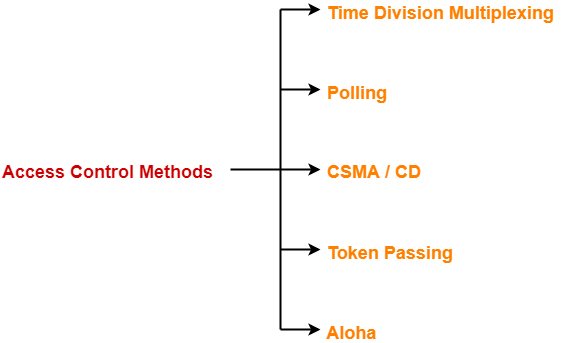In this article, we will discuss about CSMA / CD.

## CSMA / CD-

 CSMA / CD stands for Carrier Sense Multiple Access / Collision Detection.

This access control method works as follows-

## Step-01: Sensing the Carrier-

• Any station willing to transmit the data senses the carrier.
• If it finds the carrier free, it starts transmitting its data packet otherwise not.

## How?

• Each station can sense the carrier only at its point of contact with the carrier.
• It is not possible for any station to sense the entire carrier.
• Thus, there is a huge possibility that a station might sense the carrier free even when it is actually not.

## Example-

Consider the following scenario-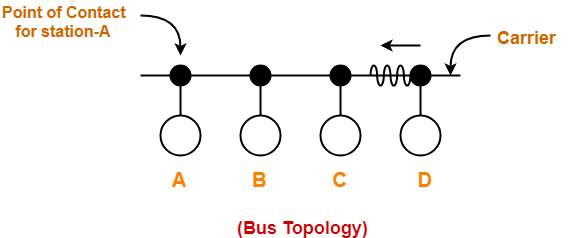At the current instance,

• If station A senses the carrier at its point of contact, then it will find the carrier free.
• But the carrier is actually not free because station D is already transmitting its data.
• If station A starts transmitting its data now, then it might lead to a collision with the data transmitted by station D.

## Step-02: Detecting the Collision-

In CSMA / CD,

• It is the responsibility of the transmitting station to detect the collision.
• For detecting the collision, CSMA / CD implements the following condition.
• This condition is followed by each station-

 Transmission delay >= 2 x Propagation delay

## Meaning-

According to this condition,

• Each station must transmit the data packet of size whose transmission delay is at least twice its propagation delay.
• If the size of data packet is smaller, then collision detection would not be possible.

## Length Of Data Packet-

We know-

• Transmission delay = Length of data packet (L) / Bandwidth (B)
• Propagation delay = Distance between the two stations (D) / Propagation speed (V)

Substituting values in the above condition, we get-

L / B >= 2 x D / V

Thus,

 L >= 2 x B x D / V

### Understanding the Condition To Detect Collision With Example

• Consider at time 10:00 am, station A senses the carrier.
• It finds the carrier free and starts transmitting its data packet to station D.
• Let the propagation delay be 1 hour.

(We are considering station D for the worst case)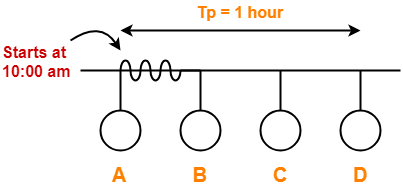• Let us consider the scenario at time 10:59:59:59 when the packet is about to reach the station D.
• At this time, station D senses the carrier.
• It finds the carrier free and starts transmitting its data packet.
• Now, as soon as station D starts transmitting its data packet, a collision occurs with the data packet of station A at time 11:00 am.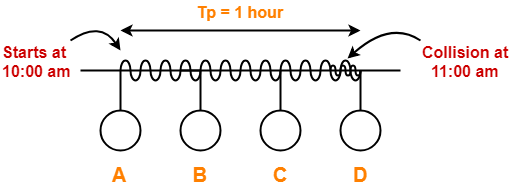• After collision occurs, the collided signal starts travelling in the backward direction.
• The collided signal takes 1 hour to reach the station A after the collision has occurred.
• For station A to detect the collided signal, it must be still transmitting the data.
• So, transmission delay of station A must be >= 1 hour + 1 hour >= 2 hours to detect the collision.
• That is why, for detecting the collision, condition is Tt >= 2Tp.

Two cases are possible-

### Case-01:

If no collided signal comes back during the transmission,

• It indicates that no collision has occurred.
• The data packet is transmitted successfully.

### Case-02:

If the collided signal comes back during the transmission,

• It indicates that the collision has occurred.
• The data packet is not transmitted successfully.
• Step-03 is followed.

## Step-03: Releasing Jam Signal-

• Jam signal is a 48 bit signal.
• It is released by the transmitting stations as soon as they detect a collision.
• It alerts the other stations not to transmit their data immediately after the collision.
• Otherwise, there is a possibility of collision again with the same data packet.
• Ethernet sends the jam signal at a frequency other than the frequency of data signals.
• This ensures that jam signal does not collide with the data signals undergone collision.

## Step-04: Waiting For Back Off Time-

• After the collision, the transmitting station waits for some random amount of time called as back off time.
• After back off time, it tries transmitting the data packet again.
• If again the collision occurs, then station again waits for some random back off time and then tries again.
• The station keeps trying until the back off time reaches its limit.
• After the limit is reached, station aborts the transmission.
• Back off time is calculated using Back Off Algorithm.

## CSMA / CD Flowchart-

The following CSMA / CD flowchart represents the CSMA / CD procedure-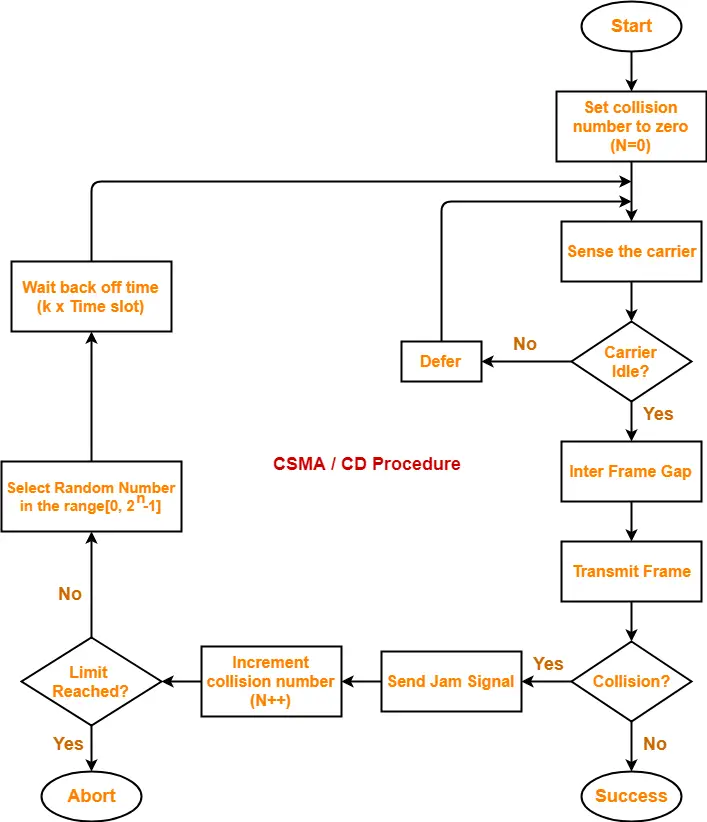## Efficiency-

 Efficiency (η) = Useful Time / Total Time

Before a successful transmission,

• There may occur many number of collisions.
• 2 x Tp time is wasted during each collision.

Thus,

• Useful time = Transmission delay of data packet = Tt
• Useless time = Time wasted during collisions + Propagation delay of data packet = c x 2 x Tp + Tp
• Here, c = Number of contention slots / collision slots.

Thus,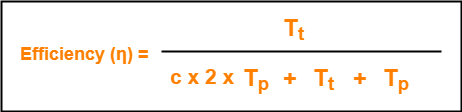Here,

• c is a variable.
• This is because number of collisions that might occur before a successful transmission are variable.

Probabilistic Analysis shows-

 Average number of collisions before a successful transmission = e

Substituting c = e in the above relation, we get-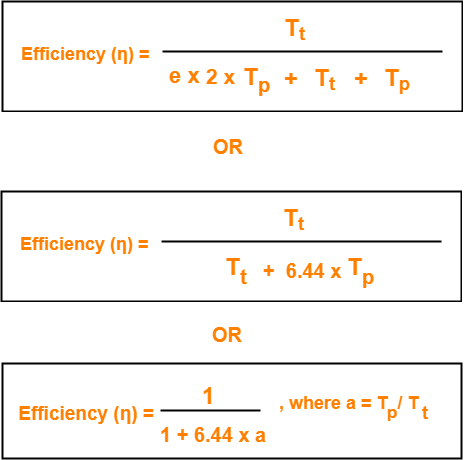## Probabilistic Analysis-

Let us perform the probabilistic analysis to find the average number of collisions before a successful transmission.

Consider-

• Number of stations connected to a CSMA / CD network = n
• Probability of each station to transmit the data = p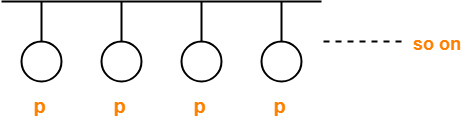Transmission will be successful only when-

• One station transmits the data
• Other (n-1) stations do not transmit the data.

Thus, Probability of successful transmission is given by-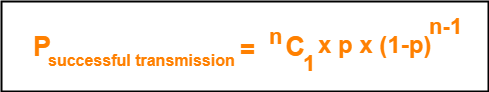Now, let us find the maximum value of Psuccessful transmission.

For maximum value, we put-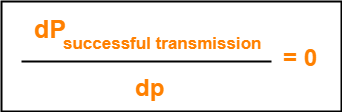On solving,

 At p = 1/n, we get the maximum value of Psuccessful transmission

Thus,

(Psuccessful transmission)max

= nC1 x 1/n x (1 – 1/n)n-1

= n x 1/n x (1 – 1/n)n-1

= (1 – 1/n)n-1

 (Psuccessful transmission)max = (1 – 1/n)n-1

If there are sufficiently large number of stations i.e. n → ∞, then we have-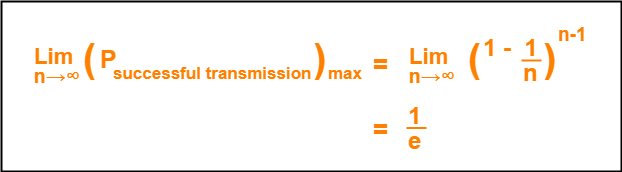Number of times a station must try before successfully transmitting the data packet

= 1 / Pmax (Using Poisson’s distribution)

= 1 / (1/e)

= e

From here, we conclude-

Average number of collisions that might occur before a successful transmission = e

## Note-01:

• CSMA / CD is used in wired LANs.
• CSMA / CD is standardized in IEEE 802.3

## Note-02:

• CSMA / CD only minimizes the recovery time.
• It does not take any steps to prevent the collision until it has taken place.

## Important Formulas-

• Condition to detect collision: Transmission delay >= 2 x Propagation delay
• Minimum length of data packets in CSMA / CD = 2 x Bandwidth x Distance / Speed
• Efficiency of CSMA / CD = 1 / (1 + 6.44 x a) where a = Tp / Tt
• Probability of successful transmission = nC1 x p x (1-p)n-1
• Average number of collisions before a successful transmission = e

To gain better understanding about CSMA / CD,

Watch this Video Lecture

Next Article- Binary Exponential Back Off Algorithm

Get more notes and other study material of Computer Networks.

Watch video lectures by visiting our YouTube channel LearnVidFun.

SummaryArticle Name
CSMA CD Protocol | CSMA CD Flowchart
Description
CSMA / CD protocol stands for Carrier Sense Multiple Access / Collision Detection. CSMA / CD in computer networks is an access control method. CSMA / CD allows the stations to sense the carrier and send the data if it is free.
Author
Publisher Name
Gate Vidyalay
Publisher Logo